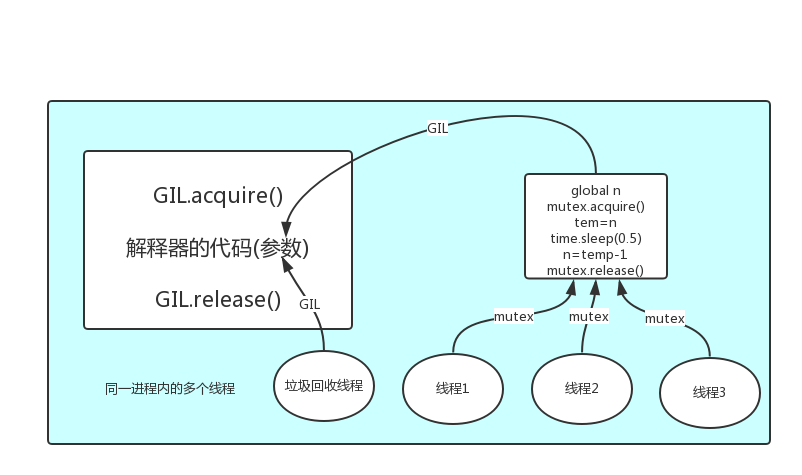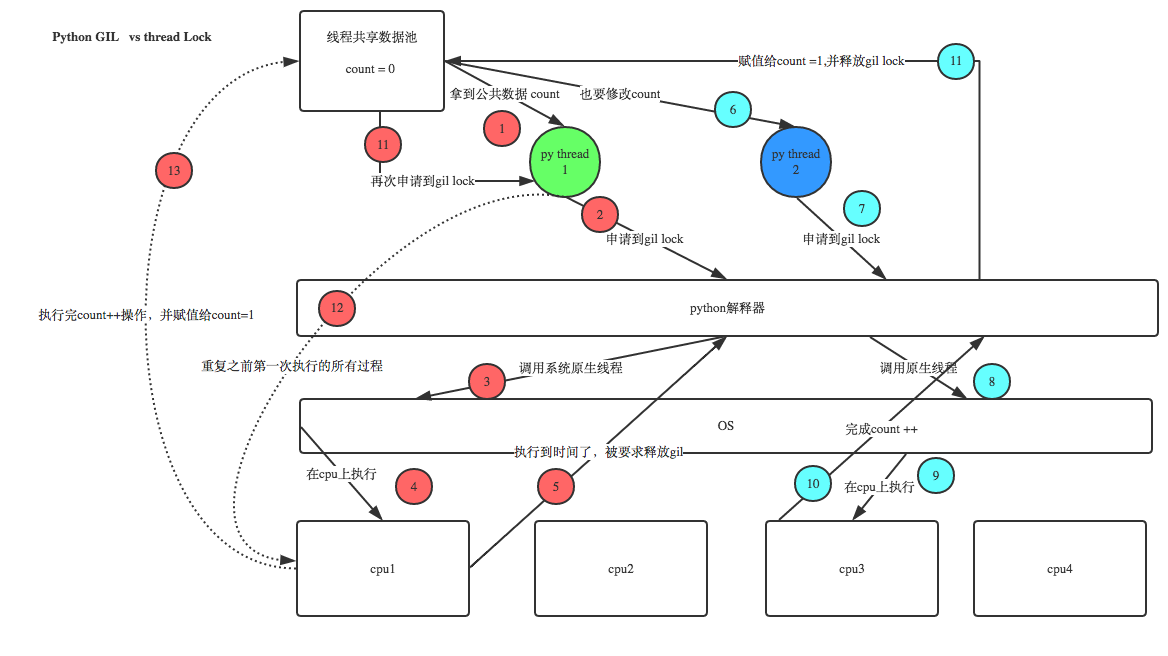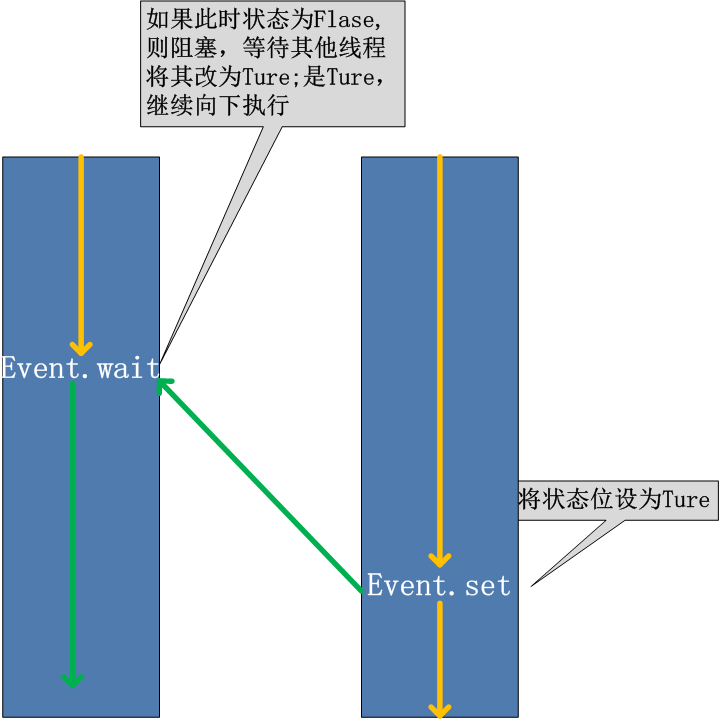目錄

一 開啟執行緒的兩種方式

#方式一
import time
def sayhi(name):
time.sleep(2)
print('%s say hello' %name)

if __name__ == '__main__':
t.start()
print('主執行緒')

#方式二
import time
def __init__(self,name):
super().__init__()
self.name=name
def run(self):
time.sleep(2)
print('%s say hello' % self.name)

if __name__ == '__main__':
t = Sayhi('egon')
t.start()
print('主執行緒')

二 在一個程序下開啟多個執行緒與在一個程序下開啟多個子程序的區別

from multiprocessing import Process
import os
def work():
global n
n=0

if __name__ == '__main__':
# n=100
# p=Process(target=work)
# p.start()
# p.join()
# print('主',n) #毫無疑問子程序p已經將自己的全域性的n改成了0,但改的僅僅是它自己的,檢視父程序的n仍然為100

n=1
t.start()
t.join()
print('主',n) #檢視結果為0,因為同一程序內的執行緒之間共享程序內的資料

三 練習

#服務端
import multiprocessing

import socket
s=socket.socket(socket.AF_INET,socket.SOCK_STREAM)
s.bind(('127.0.0.1',8080))
s.listen(5)

def action(conn):
while True:
data=conn.recv(1024)
print(data)
conn.send(data.upper())

if __name__ == '__main__':

while True:

p.start()

#客戶端
import socket

s=socket.socket(socket.AF_INET,socket.SOCK_STREAM)
s.connect(('127.0.0.1',8080))

while True:
msg=input('>>: ').strip()
if not msg:continue

s.send(msg.encode('utf-8'))
data=s.recv(1024)
print(data)

msg_l=[]
format_l=[]
def talk():
while True:
msg=input('>>: ').strip()
if not msg:continue
msg_l.append(msg)

def format_msg():
while True:
if msg_l:
res=msg_l.pop()
format_l.append(res.upper())

def save():
while True:
if format_l:
with open('db.txt','a',encoding='utf-8') as f:
res=format_l.pop()
f.write('%s\n' %res)

if __name__ == '__main__':
t1.start()
t2.start()
t3.start()

四 執行緒相關的其他方法

# isAlive(): 返回執行緒是否活動的。
# getName(): 返回執行緒名。
# setName(): 設定執行緒名。

from multiprocessing import Process
import os

def work():
import time
time.sleep(3)

if __name__ == '__main__':
#在主程序下開啟執行緒
t.start()

print('主執行緒/主程序')

'''
列印結果:
主執行緒/主程序
'''

import time
def sayhi(name):
time.sleep(2)
print('%s say hello' %name)

if __name__ == '__main__':
t.start()
t.join()
print('主執行緒')
print(t.is_alive())

五 守護執行緒

#1.對主程序來說，執行完畢指的是主程序程式碼執行完畢

#2.對主執行緒來說，執行完畢指的是主執行緒所在的程序內所有非守護執行緒統統執行完畢，主執行緒才算執行完畢

#1 主程序在其程式碼結束後就已經算執行完畢了（守護程序在此時就被回收）,然後主程序會一直等非守護的子程序都執行完畢後回收子程序的資源(否則會產生殭屍程序)，才會結束，

#2 主執行緒在其他非守護執行緒執行完畢後才算執行完畢（守護執行緒在此時就被回收）。因為主執行緒的結束意味著程序的結束，程序整體的資源都將被回收，而程序必須保證非守護執行緒都執行完畢後才能結束。
import time
def sayhi(name):
time.sleep(2)
print('%s say hello' %name)

if __name__ == '__main__':
t.setDaemon(True) #必須在t.start()之前設定
t.start()

print('主執行緒')
print(t.is_alive())

六 Python GIL(Global Interpreter Lock)

GIL本質就是一把互斥鎖，既然是互斥鎖，所有互斥鎖的本質都一樣，都是將併發執行變成序列，以此來控制同一時間內共享資料只能被一個任務所修改，進而保證資料安全。

#1 所有資料都是共享的，這其中，程式碼作為一種資料也是被所有執行緒共享的（test.py的所有程式碼以及Cpython直譯器的所有程式碼）

#2 所有執行緒的任務，都需要將任務的程式碼當做引數傳給直譯器的程式碼去執行，即所有的執行緒要想執行自己的任務，首先需要解決的是能夠訪問到直譯器的程式碼。GIL保護的是直譯器級的資料，保護使用者自己的資料則需要自己加鎖處理，如下圖#1. cpu到底是用來做計算的，還是用來做I/O的？

#2. 多cpu，意味著可以有多個核並行完成計算，所以多核提升的是計算效能

#3. 每個cpu一旦遇到I/O阻塞，仍然需要等待，所以多核對I/O操作沒什麼用處

#分析：

#單核情況下，分析結果:
如果四個任務是計算密集型，沒有多核來平行計算，方案一徒增了建立程序的開銷，方案二勝
如果四個任務是I/O密集型，方案一建立程序的開銷大，且程序的切換速度遠不如執行緒，方案二勝

#多核情況下，分析結果：
如果四個任務是計算密集型，多核意味著平行計算，在python中一個程序中同一時刻只有一個執行緒執行用不上多核，方案一勝
如果四個任務是I/O密集型，再多的核也解決不了I/O問題，方案二勝

#結論：現在的計算機基本上都是多核，python對於計算密集型的任務開多執行緒的效率並不能帶來多大效能上的提升，甚至不如序列(沒有大量切換)，但是，對於IO密集型的任務效率還是有顯著提升的。

七 同步鎖

#1.執行緒搶的是GIL鎖，GIL鎖相當於執行許可權，拿到執行許可權後才能拿到互斥鎖Lock，其他執行緒也可以搶到GIL，但如果發現Lock仍然沒有被釋放則阻塞，即便是拿到執行許可權GIL也要立刻交出來

#2.join是等待所有，即整體序列，而鎖只是鎖住修改共享資料的部分，即部分序列，要想保證資料安全的根本原理在於讓併發變成序列，join與互斥鎖都可以實現，毫無疑問，互斥鎖的部分序列效率要更高

#3. 一定要看本小節最後的GIL與互斥鎖的經典分析

GIL VS Lock:

t1.start()

t1.join

t2.start()

t2.join()

import os,time
def work():
global n
temp=n
time.sleep(0.1)
n=temp-1
if __name__ == '__main__':
n=100
l=[]
for i in range(100):
l.append(p)
p.start()
for p in l:
p.join()

R.acquire()
'''

'''
R.release()
import os,time
def work():
global n
lock.acquire()
temp=n
time.sleep(0.1)
n=temp-1
lock.release()
if __name__ == '__main__':
lock=Lock()
n=100
l=[]
for i in range(100):
l.append(p)
p.start()
for p in l:
p.join()

print(n) #結果肯定為0，由原來的併發執行變成序列，犧牲了執行效率保證了資料安全

#1.100個執行緒去搶GIL鎖，即搶執行許可權
#2. 肯定有一個執行緒先搶到GIL（暫且稱為執行緒1），然後開始執行，一旦執行就會拿到lock.acquire()
#3. 極有可能執行緒1還未執行完畢，就有另外一個執行緒2搶到GIL，然後開始執行，但執行緒2發現互斥鎖lock還未被執行緒1釋放，於是阻塞，被迫交出執行許可權，即釋放GIL
#4.直到執行緒1重新搶到GIL，開始從上次暫停的位置繼續執行，直到正常釋放互斥鎖lock，然後其他的執行緒再重複2 3 4的過程

#不加鎖:併發執行,速度快,資料不安全
import os,time
global n
temp=n
time.sleep(0.5)
n=temp-1

if __name__ == '__main__':
n=100
lock=Lock()
start_time=time.time()
for i in range(100):
t.start()
t.join()

stop_time=time.time()
print('主:%s n:%s' %(stop_time-start_time,n))

'''
......

'''

#不加鎖:未加鎖部分併發執行,加鎖部分序列執行,速度慢,資料安全
import os,time
#未加鎖的程式碼併發執行
time.sleep(3)
global n
#加鎖的程式碼序列執行
lock.acquire()
temp=n
time.sleep(0.5)
n=temp-1
lock.release()

if __name__ == '__main__':
n=100
lock=Lock()
start_time=time.time()
for i in range(100):
t.start()
t.join()
stop_time=time.time()
print('主:%s n:%s' %(stop_time-start_time,n))

'''
......

'''

#有的同學可能有疑問:既然加鎖會讓執行變成序列,那麼我在start之後立即使用join,就不用加鎖了啊,也是序列的效果啊
#沒錯:在start之後立刻使用jion,肯定會將100個任務的執行變成序列,毫無疑問,最終n的結果也肯定是0,是安全的,但問題是
#start後立即join:任務內的所有程式碼都是序列執行的,而加鎖,只是加鎖的部分即修改共享資料的部分是序列的
#單從保證資料安全方面,二者都可以實現,但很明顯是加鎖的效率更高.
import os,time
time.sleep(3)
global n
temp=n
time.sleep(0.5)
n=temp-1

if __name__ == '__main__':
n=100
lock=Lock()
start_time=time.time()
for i in range(100):
t.start()
t.join()
stop_time=time.time()
print('主:%s n:%s' %(stop_time-start_time,n))

'''
......

八 死鎖現象與遞迴鎖

import time
mutexA=Lock()
mutexB=Lock()

def run(self):
self.func1()
self.func2()
def func1(self):
mutexA.acquire()
print('\033[41m%s 拿到A鎖\033[0m' %self.name)

mutexB.acquire()
print('\033[42m%s 拿到B鎖\033[0m' %self.name)
mutexB.release()

mutexA.release()

def func2(self):
mutexB.acquire()
print('\033[43m%s 拿到B鎖\033[0m' %self.name)
time.sleep(2)

mutexA.acquire()
print('\033[44m%s 拿到A鎖\033[0m' %self.name)
mutexA.release()

mutexB.release()

if __name__ == '__main__':
for i in range(10):
t.start()

'''

'''

九 訊號量Semaphore

Semaphore管理一個內建的計數器，

import time
# def func():
#     if sm.acquire():
#         time.sleep(2)
#         sm.release()
def func():
sm.acquire()
time.sleep(3)
sm.release()
if __name__ == '__main__':
sm=Semaphore(5)
for i in range(23):
t.start()

十 Event

event.isSet()：返回event的狀態值；

event.wait()：如果 event.isSet()==False將阻塞執行緒；

event.set()： 設定event的狀態值為True，所有阻塞池的執行緒啟用進入就緒狀態， 等待作業系統排程；

event.clear()：恢復event的狀態值為False。import time,random
def conn_mysql():
count=1
while not event.is_set():
if count > 3:
raise TimeoutError('連結超時')
event.wait(0.5)
count+=1

def check_mysql():
time.sleep(random.randint(2,4))
event.set()
if __name__ == '__main__':
event=Event()

conn1.start()
conn2.start()
check.start()

十一 條件Condition

def run(n):
con.acquire()
con.wait()
con.release()

if __name__ == '__main__':

for i in range(10):
t.start()

while True:
inp = input('>>>')
if inp == 'q':
break
con.acquire()
con.notify(int(inp))
con.release()
----------------------------------------
def condition_func():

ret = False
inp = input('>>>')
if inp == '1':
ret = True

return ret

def run(n):
con.acquire()
con.wait_for(condition_func)
con.release()

if __name__ == '__main__':

for i in range(10):
t.start()

十二 定時器

def hello():
print("hello, world")

t = Timer(1, hello)
t.start()  # after 1 seconds, "hello, world" will be printed
------------------------------------------------------------------------------------
import random,time

class Code:
def __init__(self):
self.make_cache()

def make_cache(self,interval=5):
self.cache=self.make_code()
print(self.cache)
self.t=Timer(interval,self.make_cache)
self.t.start()

def make_code(self,n=4):
res=''
for i in range(n):
s1=str(random.randint(0,9))
s2=chr(random.randint(65,90))
res+=random.choice([s1,s2])
return res

def check(self):
while True:
inp=input('>>: ').strip()
if inp.upper() ==  self.cache:
print('驗證成功',end='\n')
self.t.cancel()
break

if __name__ == '__main__':
obj=Code()
obj.check()

十三 執行緒queue

queue佇列 ：使用import queue，用法與程序Queue一樣

queue is especially useful in threaded programming when information must be exchanged safely between multiple threads.

class queue.Queue(maxsize=0) #先進先出

import queue

q=queue.Queue()
q.put('first')
q.put('second')
q.put('third')

print(q.get())
print(q.get())
print(q.get())

first
second
third

class queue.LifoQueue(maxsize=0) #last in fisrt out

import queue

q=queue.LifoQueue()
q.put('first')
q.put('second')
q.put('third')

print(q.get())
print(q.get())
print(q.get())

third
second
first

class queue.PriorityQueue(maxsize=0) #儲存資料時可設定優先順序的佇列

import queue

q=queue.PriorityQueue()
#put進入一個元組,元組的第一個元素是優先順序(通常是數字,也可以是非數字之間的比較),數字越小優先順序越高
q.put((20,'a'))
q.put((10,'b'))
q.put((30,'c'))

print(q.get())
print(q.get())
print(q.get())

(10, 'b')
(20, 'a')
(30, 'c')

十四 Python標準模組--concurrent.futures

#1 介紹
concurrent.futures模組提供了高度封裝的非同步呼叫介面
ProcessPoolExecutor: 程序池，提供非同步呼叫
Both implement the same interface, which is defined by the abstract Executor class.

#2 基本方法
#submit(fn, *args, **kwargs)

#map(func, *iterables, timeout=None, chunksize=1)

#shutdown(wait=True)

wait=True，等待池內所有任務執行完畢回收完資源後才繼續
wait=False，立即返回，並不會等待池內的任務執行完畢

submit和map必須在shutdown之前

#result(timeout=None)

ProcessPoolExecutor

#用法

import os,time,random
print('%s is runing' %os.getpid())
time.sleep(random.randint(1,3))
return n**2

if __name__ == '__main__':

executor=ProcessPoolExecutor(max_workers=3)

futures=[]
for i in range(11):
futures.append(future)
executor.shutdown(True)
print('+++>')
for future in futures:
print(future.result())

map

import os,time,random
print('%s is runing' %os.getpid())
time.sleep(random.randint(1,3))
return n**2

if __name__ == '__main__':

# for i in range(11):

from multiprocessing import Pool
import requests
import json
import os

def get_page(url):
print('<程序%s> get %s' %(os.getpid(),url))
respone=requests.get(url)
if respone.status_code == 200:
return {'url':url,'text':respone.text}

def parse_page(res):
res=res.result()
print('<程序%s> parse %s' %(os.getpid(),res['url']))
parse_res='url:<%s> size:[%s]\n' %(res['url'],len(res['text']))
with open('db.txt','a') as f:
f.write(parse_res)

if __name__ == '__main__':
urls=[
'https://www.baidu.com',
'https://www.python.org',
'https://www.openstack.org',
'https://help.github.com/',
'http://www.sina.com.cn/'
]

# p=Pool(3)
# for url in urls:
#     p.apply_async(get_page,args=(url,),callback=pasrse_page)
# p.close()
# p.join()

p=ProcessPoolExecutor(3)
for url in urls: